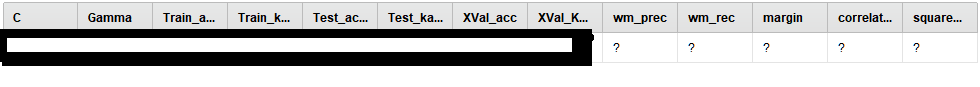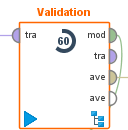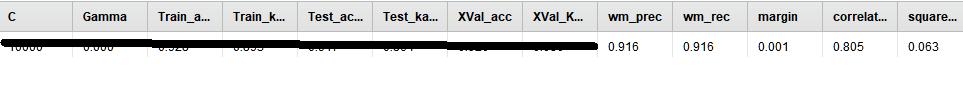# Problem logging Performance criteria or generated values in X-Validation

Member Posts: 344Unicorn
edited November 2018 in Help

hi,

I have a problem with the new "Cross Validation" Operator to log certain values from the Performance (Classification) operator that is inside that CV Operator.. I want to log weighted mean precision and recall and several other valuies like margin, etc... see the question marks in screenshot:however, in some old processes, I have stil the "old" Validation (X-Validation)  operator that does not exist anymore today in Rapidminer:and with that operator, I dont get "???" as it works:is it possible to somehow fix the new "Cross Validation" Operator in rapidminer for all the performance (Classification) criteria? (Maybe its also the same with other performance operators, didn't try it out yet..)

• Employee, Member Posts: 144RM Engineering
Solution Accepted

Hi!

Could you attach a sample process? When I try to log inside the CV operator, it works just fine. There was a minor patch for performance criterions in 7.5.3, so maybe that is the same root cause. Which version are you using?

Cheers

• Employee, Member Posts: 144RM Engineering
Solution Accepted

I see now where the problem is.Since the new cross validation is able to work in parallel, the inner performance operator does not hold the performance value. With the old validation (or when deavtivating parallelization), you would get only the last iterations performance. You could now either do the logging inside the CV (and get a sample for each iteration) or apply the "Performance to Data" operator on the performance output of the CV to get the average performance as an example set.

Here is the the modified xml with both approaches:

`<?xml version="1.0" encoding="UTF-8"?><process version="7.5.003">  <context>    <input/>    <output/>    <macros/>  </context>  <operator activated="true" class="process" compatibility="7.5.003" expanded="true" name="Process">    <process expanded="true">      <operator activated="true" class="retrieve" compatibility="7.5.003" expanded="true" height="68" name="Retrieve Iris" width="90" x="179" y="187">        <parameter key="repository_entry" value="//Samples/data/Iris"/>      </operator>      <operator activated="true" class="concurrency:cross_validation" compatibility="7.5.003" expanded="true" height="145" name="Cross Validation" width="90" x="380" y="136">        <parameter key="enable_parallel_execution" value="false"/>        <process expanded="true">          <operator activated="true" class="support_vector_machine_libsvm" compatibility="7.5.003" expanded="true" height="82" name="SVM" width="90" x="246" y="34">            <list key="class_weights"/>          </operator>          <connect from_port="training set" to_op="SVM" to_port="training set"/>          <connect from_op="SVM" from_port="model" to_port="model"/>          <portSpacing port="source_training set" spacing="0"/>          <portSpacing port="sink_model" spacing="0"/>          <portSpacing port="sink_through 1" spacing="0"/>        </process>        <process expanded="true">          <operator activated="true" class="apply_model" compatibility="7.5.003" expanded="true" height="82" name="Apply Model" width="90" x="112" y="34">            <list key="application_parameters"/>          </operator>          <operator activated="true" class="performance_classification" compatibility="7.5.003" expanded="true" height="82" name="Performance" width="90" x="246" y="34">            <parameter key="kappa" value="true"/>            <parameter key="weighted_mean_recall" value="true"/>            <parameter key="weighted_mean_precision" value="true"/>            <parameter key="relative_error" value="true"/>            <parameter key="relative_error_strict" value="true"/>            <parameter key="normalized_absolute_error" value="true"/>            <parameter key="root_mean_squared_error" value="true"/>            <parameter key="margin" value="true"/>            <list key="class_weights"/>          </operator>          <operator activated="true" class="log" compatibility="7.5.003" expanded="true" height="68" name="Log" width="90" x="179" y="136">            <list key="log">              <parameter key="a" value="operator.Performance.value.accuracy"/>              <parameter key="b" value="operator.Performance.value.weighted_mean_precision"/>              <parameter key="c" value="operator.Performance.value.weighted_mean_recall"/>              <parameter key="e" value="operator.Performance.value.margin"/>            </list>          </operator>          <connect from_port="model" to_op="Apply Model" to_port="model"/>          <connect from_port="test set" to_op="Apply Model" to_port="unlabelled data"/>          <connect from_op="Apply Model" from_port="labelled data" to_op="Performance" to_port="labelled data"/>          <connect from_op="Performance" from_port="performance" to_port="performance 1"/>          <connect from_op="Performance" from_port="example set" to_port="test set results"/>          <portSpacing port="source_model" spacing="0"/>          <portSpacing port="source_test set" spacing="0"/>          <portSpacing port="source_through 1" spacing="0"/>          <portSpacing port="sink_test set results" spacing="0"/>          <portSpacing port="sink_performance 1" spacing="0"/>          <portSpacing port="sink_performance 2" spacing="0"/>        </process>      </operator>      <operator activated="true" class="performance_to_data" compatibility="7.5.003" expanded="true" height="82" name="Performance to Data" width="90" x="514" y="187"/>      <connect from_op="Retrieve Iris" from_port="output" to_op="Cross Validation" to_port="example set"/>      <connect from_op="Cross Validation" from_port="performance 1" to_op="Performance to Data" to_port="performance vector"/>      <connect from_op="Performance to Data" from_port="example set" to_port="result 1"/>      <portSpacing port="source_input 1" spacing="0"/>      <portSpacing port="sink_result 1" spacing="0"/>      <portSpacing port="sink_result 2" spacing="0"/>    </process>  </operator></process>`

Cheers

• Member Posts: 344Unicorn
`<?xml version="1.0" encoding="UTF-8"?><process version="7.5.003">  <context>    <input/>    <output/>    <macros/>  </context>  <operator activated="true" class="process" compatibility="7.5.003" expanded="true" name="Process">    <process expanded="true">      <operator activated="true" class="retrieve" compatibility="7.5.003" expanded="true" height="68" name="Retrieve Iris" width="90" x="179" y="187">        <parameter key="repository_entry" value="//Samples/data/Iris"/>      </operator>      <operator activated="true" class="concurrency:cross_validation" compatibility="7.5.003" expanded="true" height="145" name="Cross Validation" width="90" x="380" y="136">        <process expanded="true">          <operator activated="true" class="support_vector_machine_libsvm" compatibility="7.5.003" expanded="true" height="82" name="SVM" width="90" x="246" y="34">            <list key="class_weights"/>          </operator>          <connect from_port="training set" to_op="SVM" to_port="training set"/>          <connect from_op="SVM" from_port="model" to_port="model"/>          <portSpacing port="source_training set" spacing="0"/>          <portSpacing port="sink_model" spacing="0"/>          <portSpacing port="sink_through 1" spacing="0"/>        </process>        <process expanded="true">          <operator activated="true" class="apply_model" compatibility="7.5.003" expanded="true" height="82" name="Apply Model" width="90" x="112" y="34">            <list key="application_parameters"/>          </operator>          <operator activated="true" class="performance_classification" compatibility="7.5.003" expanded="true" height="82" name="Performance" width="90" x="246" y="34">            <parameter key="kappa" value="true"/>            <parameter key="weighted_mean_recall" value="true"/>            <parameter key="weighted_mean_precision" value="true"/>            <parameter key="relative_error" value="true"/>            <parameter key="relative_error_strict" value="true"/>            <parameter key="normalized_absolute_error" value="true"/>            <parameter key="root_mean_squared_error" value="true"/>            <parameter key="margin" value="true"/>            <list key="class_weights"/>          </operator>          <connect from_port="model" to_op="Apply Model" to_port="model"/>          <connect from_port="test set" to_op="Apply Model" to_port="unlabelled data"/>          <connect from_op="Apply Model" from_port="labelled data" to_op="Performance" to_port="labelled data"/>          <connect from_op="Performance" from_port="performance" to_port="performance 1"/>          <connect from_op="Performance" from_port="example set" to_port="test set results"/>          <portSpacing port="source_model" spacing="0"/>          <portSpacing port="source_test set" spacing="0"/>          <portSpacing port="source_through 1" spacing="0"/>          <portSpacing port="sink_test set results" spacing="0"/>          <portSpacing port="sink_performance 1" spacing="0"/>          <portSpacing port="sink_performance 2" spacing="0"/>        </process>      </operator>      <operator activated="true" class="log" compatibility="7.5.003" expanded="true" height="82" name="Log" width="90" x="581" y="187">        <list key="log">          <parameter key="a" value="operator.Performance.value.accuracy"/>          <parameter key="b" value="operator.Performance.value.weighted_mean_precision"/>          <parameter key="c" value="operator.Performance.value.weighted_mean_recall"/>          <parameter key="d" value="operator.Cross Validation.value.performance 1"/>          <parameter key="e" value="operator.Performance.value.margin"/>        </list>      </operator>      <connect from_op="Retrieve Iris" from_port="output" to_op="Cross Validation" to_port="example set"/>      <connect from_op="Cross Validation" from_port="model" to_port="result 1"/>      <connect from_op="Cross Validation" from_port="performance 1" to_op="Log" to_port="through 1"/>      <connect from_op="Log" from_port="through 1" to_port="result 2"/>      <portSpacing port="source_input 1" spacing="0"/>      <portSpacing port="sink_result 1" spacing="0"/>      <portSpacing port="sink_result 2" spacing="0"/>      <portSpacing port="sink_result 3" spacing="0"/>    </process>  </operator></process>`